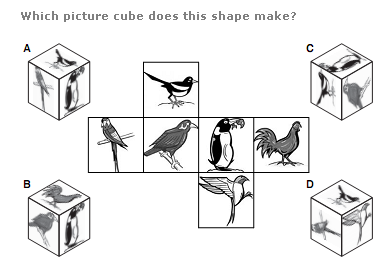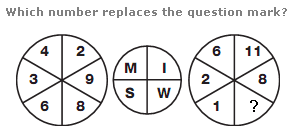# Puzzles - Logical puzzles

### Exercise :: Logical puzzlesAnswer : C Explanation : [NIL]Answer : 4 Explanation : Split the left and right hand circles into 2 halves vertically. The numerical value of the letter in the upper left segment of the central circle equals the sum of the numbers in the left half of the left hand circle, and the letter in the lower left equals the sum of the numbers in the right half of the left hand circle. Repeat this pattern for the right hand circle.Answer : 3 Explanation : Starting on the left and working to the right, take pairs of dominoes and calculate the sum of the dots they are displaying. This sum follows the sequence 3, 6, 9 and 12.